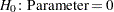### Estimated Regression Coefficients

PROC SURVEYREG  displays the "Estimated Regression Coefficients" table by default when there is no CLASS statement. Also, the procedure displays this table when you specify a CLASS statement and also specify the SOLUTION option in the MODEL statement. This table contains the following information for each regression parameter:

• Parameter, which identifies the effect or regressor variable

• Estimate, which is the estimate of the regression coefficient

• Standard Error, which is the standard error of the estimate

• t Value, which is the t statistic for testing• Pr > | t |, which is the two-sided significance probability corresponding to the t Value